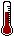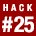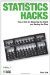# Hack 25. Predict the Future with the Normal CurveBecause almost anything we measure in the natural world has a known distributional shape, the "normal curve," we can use the precise details of that distribution to predict the future and answer all sorts of probability questions.

A variety of hacks in this book capitalize on statisticians' close personal relationship with the normal curve. "See the Shape of Everything" [Hack #23] shows how to use the normal curve to predict test performance in a general way. We can do better than that, though.

So much is known about the exact shape of this mystical curve that we can make exact predictions about the probability that scores in a certain range will be obtained. There are many other types of questions that can be asked related to test performance, and statistics can help us to answer these sorts of questions before we ever take the test!

For example:

• What are the chances that you will score between any two given scores?

• How many people will score between those two scores?

• What are the chances that you will pass your next test?

• Will you get accepted into Harvard?

• What percent of students in the U.S. will qualify as National Merit Scholars?

• What are the chances that my Uncle Frank could pass the Mensa qualifying exam?

For these types of questions, a precise tool is needed. This hack provides that tool: a table of areas under the normal curve.

#### The Table of Areas Under the Normal Curve

The normal curve is defined by the mean and standard deviation of a distribution, and the shape of the curve is always the same, regardless of what we measure, as long as the scoring system allows scores to vary. The proportions of scores falling within various areas beneath the curve, such as the space between certain standard deviations and distances from the mean, have been specified.

This hack relies on a complicated-looking table, but it is so full of useful information that it will quickly become a primary tool in your hacker's toolbox. Without further ado, take a deep breath and look at Table 3-2.

##### Table Areas under the normal curve
z scoreProportion of scores between the mean and z Proportion of scores in the larger areaProportion of scores in the smaller area
.00.00.50.50
.12.05.55.45
.25.10.60.40
.39.15.65.35
.52.20.70.30
.67.25.75.25
.84.30.80.20
1.04.35.85.15
1.28.40.90.10
1.65.45.95.05
1.96.475.975.025
4.00.501.00.00

#### Deciphering the Table

Before we use this nifty tool, we need to take a second deep breath and get the lay of the land. I have simplified the information on this table in a couple of ways. First, I have listed only a few of the values that could be computed. Indeed, many tables in statistical books have every value between a z of .00 and a z of 4.00, increasing at the rate of .01. That's a lot of information that could be presented, so I have chosen to show only a glimpse of the most commonly needed values, including the z scores necessary for 90 percent confidence (1.65) and 95 percent confidence intervals (1.96); see "Measure Precisely" [Hack #6] for more on confidence intervals.

I have also rounded the proportions to two decimal places. Finally, I used the symbol z in the table to indicate the distance from the mean in standard deviations. You can learn more about z scores in "Give Raw Scores a Makeover" [Hack #26].

After understanding the simplifications made to the table, the first step toward using it to make probability predictions about performance or answer statistical questions is to understand the four columns.

The z column

Picture the normal curve [Hack #23]. If you are interested in some score that could fall along the bottom horizontal line, it is some distance from the mean. It could be greater than the mean score or less than it. The distance to the mean expressed in standard deviations is the z score. A z score of 1.04 describes a score that is a little more than one standard deviation away from the mean. Because the normal curve is symmetrical, we don't bother to note whether the distance is negative or positive, so all of these z scores are shown as positive.

Proportion of scores between the mean and z

In that space between a given score and the mean, there will be a certain proportion of scores. This is the probability that a random score will fall in the area defined by the mean and any z.

Proportion of scores in the larger area

You could also describe the area between any given z and a z of 4.00, or the end of the curve.

The curve doesn't really ever end, theoretically, but a z score of 4.00 will come very close to including 100 percent of the scores.

There are two ends of the curve, though. Unless your z is 0.0, the distance between the z and one end of the curve will be greater than the distance between the z and the other end. This column refers to the area between the z and that furthest end of the curve, and the value in this column is the proportion of scores that will fall in that space. In other words, it is the chance that a random person will produce a score in that area.

Proportion of scores in the smaller area

This column refers to the area between the z and that closest end of the curve. It is the proportion of scores that will fall in that space.

#### Estimating the Chance of Scoring Above or Below Any Score

If you need to know your chances of getting into your college of choice, identify the necessary score you need to beat, also known as the cut score, on that school's admissions tests. Once you know the score, find out the mean and standard deviation for the test. (All of this info is probably on the Web.) Convert your raw score to a z score [Hack #26], and then find that z score, or something close to it, in Table 3-2.

Determine whether the cut score is above the mean:

• If it is, look at the "Proportion of scores in the smaller area" column. That represents your chances of scoring at or above that cut score, and your chances of getting in.

• If the cut score is below the mean (unlikely, but for the sake of completely training you on how to use this tool), identify "Proportion of scores in the larger area." That's the proportion of students being accepted and, thus, your chances, all things being equal.

For the chances of scoring below a given score, the process is the opposite of the options just mentioned. The chance of getting below a specific cut score that is below the mean is shown in the "smaller area" column. The chance of scoring below a given cut score that is above the mean is shown in the "larger area" column.

#### Estimating the Chance of Scoring Between Any Two Scores

The chances of getting a score within any range of scoringscores can be determined by looking at the proportion of scores that will normally fall in that range.

If you want to know what proportion of scores falls between any two points under the curve, define those points by their z score and figure out the relevant proportion. Depending on whether both scores fall on the same side of the mean, one of two methods will give you the correct proportion between those points:

• If the z scores are on the same side of the curve, look up the proportion of scores in either the "larger area" or "smaller area" column for both z scores and subtract the lower value from the higher value.

• If the z scores fall on both sides of the mean with the mean between them, use the "Proportion of scores between the mean and z" column. Look up the value for both scores and add them together.

#### Producing Percentile Ranks

A third use of the table is to compute percentile ranks. You can read more about such norm-referenced scores in "Produce Percentiles" [Hack #24]. For scores above the mean, the percentile rank is "Proportion of scores between the mean and z" plus .50. For scores below the mean, the percentile rank is "Proportion of scores in the smaller area."

#### Determining Statistical Significance

Another use for these sorts of tables is to assign statistical significance [Hack #4] to differences in scores. By knowing the proportion of scores that will fall a certain distance from each other or further, you can assign a statistical probability to that outcome.

More usefully, other statistical values such as correlations and proportions can be converted to z scores, and this table can be used to compare those values to zero or to each other.

#### Why It Works

"See the Shape of Everything" [Hack #23] provides a good picture of the normal curve. However, just by looking at the way these values change in Table 3-2, you can get a good sense of the normal distribution's shape. Near the mean, where the rows have smaller z scores, a goodly proportion of scores will fall. As you move further and further away from the mean, it takes larger and larger areas of the curve to contain the same proportion of scores.

For example, it takes a jump from a z of 1.65 to 4 just to cover that last 5 percent of the distribution. Near the mean, though, it requires only a jump from z = .12 to z = .25 to cover 5 percent of scores. The table demonstrates how common it is to be common and how rare it is to be scarce.

#### See Also

• You will be able to compute your own exact areas under the normal curve by using this web site: http://www.psychstat.missouristate.edu/introbook/sbk11m.htm. A good discussion and some interactive calculators are part of this site maintained by David Stockburger. When you visit, don't be confused by words like Mu and Sigma. That's stats talk for mean and standard deviation, respectively.Statistics Hacks: Tips & Tools for Measuring the World and Beating the Odds
ISBN: 0596101643
EAN: 2147483647
Year: 2004
Pages: 114
Authors: Bruce Frey

Similar book on Amazon

flylib.com © 2008-2017.
If you may any questions please contact us: flylib@qtcs.net